# Math in Focus Grade 7 Chapter 3 Lesson 3.7 Answer Key Real-World Problems: Algebraic Reasoning

Go through the Math in Focus Grade 7 Workbook Answer Key Chapter 3 Lesson 3.7 Real-World Problems: Algebraic Reasoning to finish your assignments.

## Math in Focus Grade 7 Course 2 A Chapter 3 Lesson 3.7 Answer Key Real-World Problems: Algebraic Reasoning

### Math in Focus Grade 7 Chapter 3 Lesson 3.7 Guided Practice Answer Key

Complete.
Question 1.
The area of a triangle is (u + 10) square centimeters. The ratio of the area of the unshaded region to the area of the shaded region is 1 : 3. Using algebraic reasoning, express the area of the shaded region in terms of u.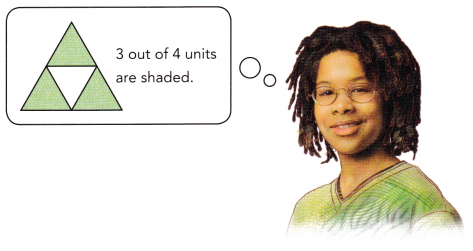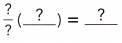Use the distributive property. Simplify.
The area of the shaded region of the triangle is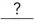square centimeters.
The area of the shaded region of the triangle is $$\frac{3}{4}$$ (u + 100) square centimeters.

Explanation:
The area of a triangle is (u + 10) square centimeters.
The ratio of the area of the unshaded region to the area of the shaded region is 1 : 3.
Area of the shaded region of the triangle = $$\frac{3}{4}$$ × Area of a triangle
= $$\frac{3}{4}$$ ×(u + 10)
= $$\frac{3}{4}$$ (u + 100) square centimeters.

Question 2.
There are 25 nickels and quarters, w coins are nickels and the rest are quarters.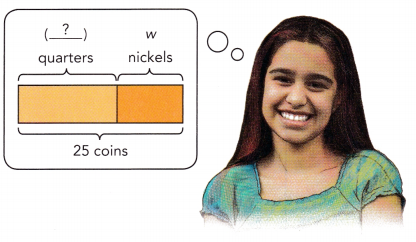a) Write an algebraic expression for the number of coins that are quarters.
Number of quarters: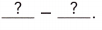There are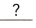quarters.
Number of quarters = 25 – w.

Explanation:
There are 25 nickels and quarters,
w coins are nickels and the rest are quarters.
=> Total number of coins = 25.
=> Number of nickels = w.
Number of quarters = Total number of coins – Number of nickels
= 25 – w.

b) Find the total value of the quarters.
Total value of quarters: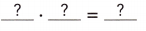The total value of the quarters is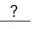dollars.
Total value of quarters = (25 – w) dollars.

Explanation:
Number of quarters = 25 – w.
Total value of quarters = (25 – w) dollars.

Complete each ? with + or -, and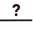with the correct value.
Question 3.
Amy has x comic books, Melvin has $$\left(\frac{2 x}{5}+1\right)$$ comic books, and Joel has $$\frac{x}{10}$$ fewer comic books than Melvin. Express the total number of comic books that Amy, Melvin, and Joel have in terms of x.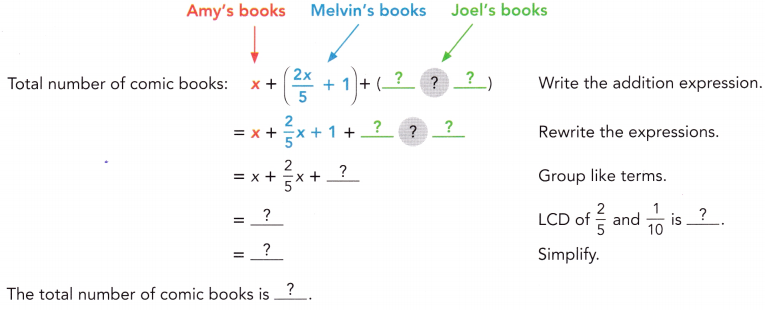Total number of comic books = $$\frac{17x}{10}$$ + 2.

Explanation:
Amy has x comic books, Melvin has $$\left(\frac{2 x}{5}+1\right)$$ comic books, and Joel has $$\frac{x}{10}$$ fewer comic books than Melvin.
Number of Melvin has comic books = $$\left(\frac{2 x}{5}+1\right)$$
Number of Joel has comic books = Number of Melvin has comic books – $$\frac{x}{10}$$
=> $$\left(\frac{2 x}{5}+1\right)$$ – $$\frac{x}{10}$$
=>$$\frac{2x}{5}$$ – $$\frac{x}{10}$$ + 1
LCD of 5 n 10 = 10.
=> (2x × 2) – x] ÷ 10 + 1
=> (4x -x) ÷ 10 + 1
=> $$\frac{3x}{10}$$ + 1.
Total number of comic books = Number of  Amy has comic books + Number of Melvin has comic books + Number of Joel has comic books
= x + $$\left(\frac{2 x}{5}+1\right)$$  + $$\frac{3x}{10}$$ + 1
= x + $$\frac{2x}{5}$$ + $$\frac{3x}{10}$$ + 1 + 1
LCD of 5 n 10 = 10.
= [10x + (2x × 2) + 3x] ÷ 10 + 2
= (10x + 4x + 3x) ÷ 10 + 2
= $$\frac{17x}{10}$$ + 2.

Solve.
Question 4.
A shoe store stocks x pairs of sneakers and y pairs of sandals. During a promotion, a pair of sneakers is priced at $50 and a pair of sandals at$36. The shop manages to sell half the sneakers and 80% of the sandals. Write an expression for the total amount of sales the store makes.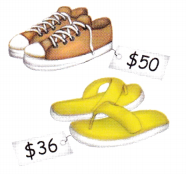Total amount of sales the store makes = $$\frac{x}{2}$$ + $$\frac{4}{5}$$y.

Explanation:
A shoe store stocks x pairs of sneakers and y pairs of sandals.
Total number of pair of sneakers a shoe store = x.
Total number of pair of sandals a shoe store = y.
a pair of sneakers is priced at $50 and a pair of sandals at$36.
=> Cost of pair of sneakers = $50. Cost of pair of sandals =$36.
The shop manages to sell half the sneakers and 80% of the sandals.
Number of sneakers sold = Total number of pair of sneakers a shoe store ÷ 2.
= x ÷ 2 or $$\frac{x}{2}$$
Number of sandals sold = (80 ÷ 100) × y
= $$\frac{80}{100}$$y.
= $$\frac{4}{5}$$y
Total amount of sales the store makes = Number of sneakers sold + Number of sandals sold
= $$\frac{x}{2}$$ + $$\frac{4}{5}$$y.

### Math in Focus Course 2A Practice 3.7 Answer Key

Solve each question using algebraic reasoning.
Question 1.
40% of k liters of acid are added to 60% of w liters of water. Write an algebraic expression for the total volume of the solution.
Total volume of the solution = (40% × k) + (60% × w).

Explanation:
40% of k liters of acid are added to 60% of w liters of water.
=> (40% × k) + (60% × w)
Total volume of the solution = (40% × k) + (60% × w).

Question 2.
Write an algebraic expression for the perimeter of the quadrilateral shown.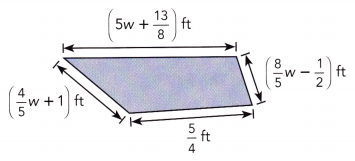Perimeter of the quadrilateral = $$\frac{37}{5}$$w + $$\frac{17}{8}$$

Explanation:
Perimeter of the quadrilateral = AB + BC + CD + AC
Perimeter of the quadrilateral = [5w + $$\frac{13}{8}$$] ft + [$$\frac{8}{5}$$w – $$\frac{1}{2}$$] ft +  $$\frac{5}{4}$$ ft + [$$\frac{4}{5}$$w + 1] ft
= 5w + $$\frac{8}{5}$$w + $$\frac{4}{5}$$w + $$\frac{13}{8}$$ – $$\frac{1}{2}$$ + 1
LCD of 8 n 2 = 8.
= [(25w + 8w + 4w) ÷ 5] + [(13 – (1 × 4) + 8) ÷ 8]
= (33w + 4w) ÷ 5] + (13 – 4 + 8) ÷ 8
= (37w ÷ 5) + [(9 + 8) ÷ 8]
= (37w ÷ 5) + (17 ÷ 8) or $$\frac{37}{5}$$w + $$\frac{17}{8}$$

Question 3.
If the average daily sales amount for the past 5 days was (2.3q + 1.4) dollars, write an algebraic expression for the total sales amount for the past 5 days.
Total sales amount for the past 5 days = 4.6q + 2.8.

Explanation:
If the average daily sales amount for the past 5 days was (2.3q + 1.4) dollars.
=> Let the sales be x.
Average daily sales for 5 days = (2.3q + 1.4)
Total sales amount for the past 5 days = Average daily sales for 5 days × 2
= (2.3q + 1.4) × 2
= (2.3q × 2) + (1.4 × 2)
= 4.6q + 2.8.

Question 4.
The weight of 2 science fiction books and 1 autobiography is $$\frac{5}{6}$$ w pounds. What is the total weight of 4 of these science fiction books and 2 of these autobiographies?
Total weight of 4 of these science fiction books and 2 of these autobiographies = $$\frac{5}{3}$$ w pounds.

Explanation:
The weight of 2 science fiction books and 1 autobiography is $$\frac{5}{6}$$ w pounds.
=> Weight of 2 science fiction books and 1 autobiography = $$\frac{5}{6}$$ w pounds.
Total weight of 4 of these science fiction books and 2 of these autobiographies = 2 × Weight of 2 science fiction books and 1 autobiography
= 2 × $$\frac{5}{6}$$ w pounds.
= $$\frac{5}{3}$$ w pounds.

Question 5.
On her way to work, Ms. Bowman waited 20 minutes at a subway station. The train ride took her (x + 30) minutes to reach Grand Central Station. Then, she walked for another $$\frac{1}{3}$$x minutes before reaching her office. How much time, in minutes, did Ms. Bowman take to travel to her office?
Number of minutes Ms. Bowman take to travel to her office = $$\frac{4}{3}$$x + 50.

Explanation:
Number of minutes Ms. Bowman waited  at a subway station = 20.
Number of minutes train ride took her to reach Grand Central Station = (x + 30)
Number of minutes train she walked before reaching her office = $$\frac{1}{3}$$x
Number of minutes Ms. Bowman take to travel to her office = Number of minutes Ms. Bowman waited  at a subway station + Number of minutes train ride took her to reach Grand Central Station + Number of minutes train she walked before reaching her office
= 20 + (x + 30) + $$\frac{1}{3}$$x
= x + 20 + 30 + $$\frac{1}{3}$$x
= x + $$\frac{1}{3}$$x + 50
= [(3x + x) ÷ 3] + 50
= $$\frac{4}{3}$$x + 50.

Question 6.
A ribbon measuring (v + 4) feet in length was cut into two pieces in the ratio 3 : 7. What was the length of the longer piece?
Length of longer piece =  7x or 7(v+4)/10.

Explanation:
Total length of ribbon = (v + 4)
Ratio of the ribbon cut into two pieces = 3 : 7
=>let the two pieces be 3x and 7x , then
3x + 7x = v+4
10x = v+4
x = (v+4)/10
Length of the two pieces:
one piece = 3x or 3(v+4)/10.
Other piece =  7x or 7(v+4)/10.

Question 7.
When one-fifth of the boys left, there were still b boys and g girls who stayed to see a program. What was the total number of boys and girls at the beginning of the program?
Total number of boys and girls at the beginning of the program = $$\frac{5}{4}$$b + g.

Explanation:
Number of girls = g.
Let the boys at the beginning be x.
Then, subtract one fifth from x to get b boys.
=> x – $$\frac{1}{5}$$ = b.
=> (5x – x) ÷ 5 = b
=> 4x = b × 5
=> 4x = 5b
=> x = $$\frac{5}{4}$$b.
Total number of boys and girls at the beginning of the program = Number of boys at the beginning + Number of girls
= $$\frac{5}{4}$$b + g.
Question 8.
John is paid at an hourly-rate of $15 an hour and overtime time rate of 1.5 times his hourly-rate for his work. If John puts in w regular hours and y overtime hours in a week, what is his total wage for the week? Answer: Total wage for the week =$262.50.

Explanation:
Number of dollars John is paid at an hourly-rate for a day= 15.
overtime time rate of 1.5 times his hourly-rate for his work.
=> Number of dollars he gets for overtime for a day = 1.5 × Number of dollars John is paid at an hourly-rate for a day
= 1.5 × 15
= 22.5.
If John puts in w regular hours and y overtime hours in a week.
Number of days in a week = 7.
Total wage for the week = Number of days in a week × (Number of dollars John is paid at an hourly-rate for a day + Number of dollars he gets for overtime for a day)
= 7  × ( $15 +$22.5)
= 7 × $37.5 =$262.50.

Question 9.
Nathan is p years old now. In 10 years’ time, he will be 3 times as old as Martin. Express Martin’s age 10 years from now in terms of p.
Martin’s age 10 years from now = (p – 20) ÷ 3 years.

Explanation:
Age of Nathan now = p years.
Let Martin age be m years now.
In 10 years’ time, he will be 3 times as old as Martin.
Age of Nathan 10 years later = (p + 10) = 3(m + 10)
=> p + 10 = 3m + 30
=> p = 3m + 30 – 10
=> p = 3m + 20.
=> p – 20 = 3m
=> (p – 20) ÷ 3 years = m.

Question 10.
Tom bought a computer for 15% off from the list price of p dollars. If the sales tax was 8%, how much did he pay for the computer including sales tax?
Total price of computer he paid= 0.918p.

Explanation:
List price of computer Tom bought = p dollars.
Tom bought a computer for 15% off
=> Sales price of computer = p – (15% × p)
= (100p – 15p) ÷ 100
= 85p ÷ 100
= 0.85p.
Tax on Sales tax = (8% ) × Sales price of computer
= (8 ÷ 100) × 0.85p
= 0.08 × 0.85p
= 0.068p.
Total price of computer he paid = Sales price of computer  + Tax on Sales tax
= 0.85p + 0.068p
= 0.918p.

Question 11.
A farmer collected some eggs from his farm and found b eggs broken. He packed the remaining eggs in c egg cartons. Each egg carton can hold a dozen eggs and no eggs were leftover. Write an algebraic expression for the number of eggs he collected initially in terms of b and c.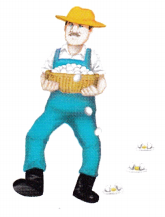Number of eggs he collected initially = 12c + b.

Explanation:
Number of eggs broken = b.
Number of remaining eggs he packed in carton = c.
Number of eggs each canton can hold = 12 or dozen.
Number of eggs he collected initially = (Number of eggs each canton can hold × Number of remaining eggs he packed in carton) + Number of eggs broken
= (12 × c) + b
= 12c + b.

Question 12.
A teacher from Anderson Middle School printed k nametags in preparation for the science fair. Half of the nametags were given out to students and 100 nametags were given out to parents. Three-fifths of the remaining nametags were given out to teachers and contest judges. How many nametags were not given out?
Number of nametags not given = $$\frac{1}{5}$$k –  40.

Explanation:
Total number of nametags teacher from Anderson Middle School printed in preparation for the science fair = k.
Number of nametags given to students = $$\frac{1}{2}$$ × Total number of nametags teacher from Anderson Middle School printed in preparation for the science fair
= $$\frac{1}{2}$$ × k
= $$\frac{1}{2}$$k.
Number of nametags given to parents = 100.
Total number of nametags given to parents and students = Number of nametags given to students + Number of nametags given to parents
= $$\frac{1}{2}$$k + 100
Remaining nametags = k – [$$\frac{1}{2}$$k + 100]
= (2k – k) ÷ 2 – 100
= $$\frac{1}{2}$$k – 100
Total number of nametags given to parents and students = $$\frac{3}{5}$$ [Remaining nametags]
= $$\frac{3}{5}$$ [$$\frac{1}{2}$$k – 100]
= $$\frac{3}{10}$$k – 60.
Number of nametags not given = $$\frac{2}{5}$$ [$$\frac{1}{2}$$k – 100]
= $$\frac{1}{5}$$k –  40.

Question 13.
At the beginning of a journey, the fuel tank of a car was $$\frac{3}{4}$$-full. When the car reached its destination, it had consumed 60% of the gasoline in the tank. The full capacity of the fuel tank is w gallons.
a) Write an algebraic expression for the amount of gasoline left in the fuel tank.
Amount of gasoline left in the fuel tank = 2w ÷ 5 gallons.

Explanation:
Total Capacity of fuel in the car = w gallons.
Capacity of the fuel tank of a car at the beginning of a journey = $$\frac{3}{4}$$w gallons.
Capacity of fuel the car consumed to reach its destination = 60% ×Total Capacity of fuel in the car
= 60% of w.
= $$\frac{6}{10}$$w
= $$\frac{3}{5}$$ w gallons.
Amount of gasoline left in the fuel tank = Total Capacity of fuel in the car – Capacity of fuel the car consumed to reach its destination
= w – $$\frac{3}{5}$$ w.
= (5w – 3w) ÷ 5
= 2w ÷ 5 gallons.

b) If w = 15.5, how much gasoline was left at the end of the journey?
Amount of gasoline left at the end of the journey = 6.2 gallons.

Explanation:
Amount of gasoline left in the fuel tank = 2w ÷ 5 gallons.
If w = 15.5:
Amount of gasoline left at the end of the journey = 2w ÷ 5
= (2 × 15.5) ÷ 5
= 31 ÷ 5
= 6.2 gallons.

Bain @ work
Bryan and his father are from Singapore, where the temperature is measured in degrees Celsius. While visiting downtown Los Angeles, Bryan saw a temperature sign that read 72°F. He asked his father what the equivalent temperature was in °C.

His father could not recall the Fahrenheit-to-Celsius conversion formula, C = $$\frac{5}{9}$$(F – 32). However, he remembered that water freezes at 0°C or 32°F and boils at 100°C or 212°F.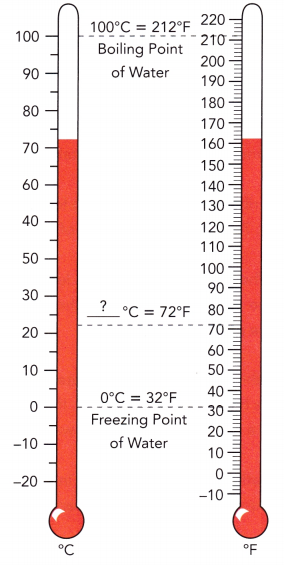Using these two pieces of information, would you be able to help Bryan figure out the above conversion formula? Explain.
(F – 32) ×$$\frac{5}{9}$$
= (72 – 32) × $$\frac{5}{9}$$
= 40 × $$\frac{5}{9}$$